Improving the Accuracy of Yoshio's Formula Koide | OMICS International
Journal of Physical Mathematics

# Improving the Accuracy of Yoshio's Formula Koide

Mareau D*

Independent Research Engineer, France

*Corresponding Author:
Mareau D
Independent Research Engineer
France
Tel: (33) 0476620296
E-mail: [email protected]

Received Date: February 22, 2016; Accepted Date: April 26, 2016; Published Date: May 03, 2016

Citation: Mareau D (2016) Improving the Accuracy of Yoshio's Formula Koide. J Phys Math 7: 168. doi: 10.4172/2090-0902.1000168

Copyright: © 2016 Mareau D. This is an open-access article distributed under the terms of the Creative Commons Attribution License, which permits unrestricted use, distribution, and reproduction in any medium, provided the original author and source are credited.

Visit for more related articles at Journal of Physical Mathematics

#### Abstract

The formula Yoshio Koide, shows a pretty compelling link between the masses of leptons: electron, muon, tauon. We offer a clear improvement in accuracy in expressing this formula electron unit. This opens the way for the assumption of heavy leptons type of composite. Under discussion is then referred to the cause, beyond the standard model, the neutrino flavor mixture, treated with PMNS matrix.

#### Keywords

Leptons; Muon; Tauon; Integers

#### Introduction

Yoshio's Formula Koide

With the following 3 leptons :

me=0,510998910(13) MeV/c² mμ=105,658367(4) MeV/c² mτ=1776,82(16) MeV/c²

We obtain this result: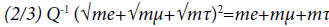(1)

With Q=1+1, 2594453 × 10-5. This law is not explained but appears quite convincing [2-11].

Test with the nearest integer

Another solution is to express the masses entire unit electron. We take the muon to determine the unique conversion ratio: τ=207/206, 76828(4)=1,001120758(4). Then adjusting the mass of the tauon with the same conversion rate: 3481 bare unit electron. Thus this number, 3477, 10274(4), related to: 1776,79584(4) MeV/c², is consistent with the measurement: 1776,82(16) MeV/c².

leptons Me V/c2 electron unit T conversion(rate) whole round
me 0,510998910(13) 1 1 1
mµ 105,658367(4) 206,768282(4) 1,001120758 207
mT 1776,79584(4) 3477,10274(4) 1,001120758 3481

One thus obtains the following result, Q=1+1, 1775 × 10-7: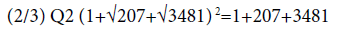(2)

This is a prediction of the mass of tauon: 1776, 79584(4) MeV/c². Is in the confidence interval of the measure: 1776, 82(16) MeV/c² . It is a falsifiable and plausible hypothesis.

#### Discussion

In the Standard Model, heavy leptons are fundamental particles. However, the result of the relationship (2), is troubling. The digital occurrence is improved by a factor >100. The only clue is not sufficient to establish a lepton composed of electron-positron pairs. But this possibility offered by integers, expressed in units electron, is intriguing. Beyond the standard model, we will look at constraints to consider a composite muon. For this, we will compare the muon in para positronium, p-Ps . The latter is composed of a single electron-positron pair. These fermions comply Fermi statistics. The fermion condensation is unstable. The lifetime of the para positronium is approximately: 10-10 s. The lifetime of the muon is approximately: 10-6 s.

This comparison should consider the following:

• p-Ps decays into photon mode while the muon decays into neutrino mode,

Muon has the exact charge of an electron,

• The two elements compared (p-Ps and muon) are unstable,

• The p-Ps is not submitted as the muon, the weak force, via boson W-.

For the muon, we make the following hypotheses:

a) It has a neutral group of 103 pairs of electrons, positrons, or 206 units,

b) This neutral group is modeled as a stack of hollow spheres of radius: Æ�μ,

c) During this short time, fermions behave as pseudo bosons,

d) The vector sum cancels masking the charges contrary superposed,

e) Muon charge is given by the single electron confined in the center,

f) The emitted neutrino, νμ represents the neutral group, in another form,

g) The antineutrino ν¯e corresponds to the differential virtual dressing constitutive pairs,

h) The differential virtual casing is the ratio of table 1: 1,001120758

i) The emitted electron is the one that was confined to the muon,

j) Neutrino states ((f-g) may be treated with the mixture of matrix PMNS.

The masking of stacked charges solves the problem of the limits of the electromagnetic force. The Æ�μ radius muon, is a function of the reduced length of the length of Compton electron, adjusted by the ratio of the masses, by: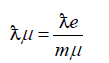(3)

Agreed: Æ�μ=1,86759 × 10-15 m, with mμ, mass (dimensionless) in electron unit (206,76828), table 1. At this level of condensation, the charges are completely masked. This is an optimal radius for beyond and below, the masking disappears. Thus the neutral part of the muon is in a wave-particle intermediate state. The electrostatic force is therefore zero. The 103 neutral pairs form a very unstable condensate. The central charge tends to polarize the neutral layers. The attempt for the electron to cross the neutral sphere, is to unmask the charges and therefore increase the internal energy. This potential wells tends to confine the electron. This spherical symmetry allows using the Gauss theorem. The field flux passing through the closed surface is equal to entire charge within the volume divided by εo.It has the general form: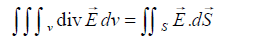(4)

with ρ, the volume charge density: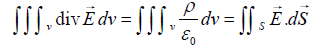(5)

In the case of the neutral sphere, the charges are canceled and: ρ=0. If the charges confined disrupts the sphere, the potential difference between two points is by integrating the electric field between these two points

According to the standard model, the lifetime of the para positronium is given by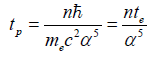(6)

With n=2 and the period (te=Æ�e/c) of the electron, tp ~ 1,42 × 10-10 s. Posing: n=5/4 × 206, we approach the lifetime of the muon, according:

<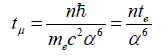(7)

With: 2,196499 × 10-6 s, for: 2,1969811(22) × 10-6 s, measured . The order 6 for the fine structure constant, is that of orthopositronium . The 5/4 ratio is a free parameter. The decay mode of tauon, 67% hadron, distinguishes muon. Calculating the lifetime of tauon is more complex. This model will remain a speculation if it is not confirmed by other independent channels. For all neutrino models, there is the following riddle: it propagates at the speed of light and oscillates between different flavors. This is inconsistent with the notion of mass. And at its disintegration, loss of mass is unexplained. By following this model of muon, one can imagine that divides to form 2 × 103 units. This opens the way for the assumption of a Majorana neutrino, where the two opposing parties are canceled. But this implies opposite actions, not scalar. However there is a constant unknown physics: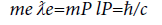(8)

It compares the product (mass × length) of the electron with the Planck. According to (3) it also applies to the muon: mμ Æ�μ. It is not trivial for these six reasons:

a. It is precise and constant, by: Ä§/c

b. It suggests that the product [M. L] is an indivisible entity

c. It Confirms the Relation (3)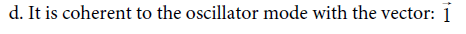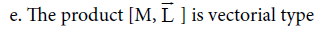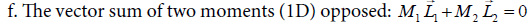According to these 6 points, the mass of a quantum particle is inseparable from its vibration amplitude. It is inversely proportional. For this variety of neutrino, the overall moment is canceled by the vector sum of the two opposing parties. This Majorana mode, and conceals 2 confined and inseparable referential. In the overall referential, the mass is null. This scenario allows to reconcile three conflicting measures: a) the oscillation of flavors; b) the propagation velocity of light; c) the unexplained disappearance of the mass after muon decay. There is an analogy with the gyroscopic moment of a rotating disk. The overall gyroscopic moment (apparent) is canceled if one has 2 discs in opposite rotations on the same axis. But the moment is preserved in the reference of each disc. The coefficients of anomalies  Magnetic moment: a) muon=1.00116592; b) electron=1.00115965, are very close. This is compatible with the confinement of an electron in the muon. The small difference is explained by the influence of the neutral party.

#### Conclusion

According to this hypothesis, at the quantum scale, the mass measurement is inseparable from its spatial amplitude vibration vector. It would therefore be measured as part scalar, the vector product. In conclusion, we must insist on the importance of the relationship 8 and his non-trivial consequences.

#### References

Select your language of interest to view the total content in your interested language

### Article Usage

• Total views: 9390
• [From(publication date):
June-2016 - Jan 18, 2020]
• Breakdown by view type
• HTML page views : 9213Can't read the image? click here to refresh